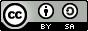# 词向量¶

## 说明¶

1. 本教程可支持在 CPU/GPU 环境下运行

2. Docker镜像支持的CUDA/cuDNN版本

如果使用了Docker运行Book，请注意：这里所提供的默认镜像的GPU环境为 CUDA 8/cuDNN 5，对于NVIDIA Tesla V100等要求CUDA 9的 GPU，使用该镜像可能会运行失败;

3. 文档和脚本中代码的一致性问题

请注意：为使本文更加易读易用，我们拆分、调整了train.py的代码并放入本文。本文中代码与train.py的运行结果一致，可直接运行train.py进行验证。

## 背景介绍¶

One-hot vector虽然自然，但是用处有限。比如，在互联网广告系统里，如果用户输入的query是“母亲节”，而有一个广告的关键词是“康乃馨”。虽然按照常理，我们知道这两个词之间是有联系的——母亲节通常应该送给母亲一束康乃馨；但是这两个词对应的one-hot vectors之间的距离度量，无论是欧氏距离还是余弦相似度(cosine similarity)，由于其向量正交，都认为这两个词毫无相关性。 得出这种与我们相悖的结论的根本原因是：每个词本身的信息量都太小。所以，仅仅给定两个词，不足以让我们准确判别它们是否相关。要想精确计算相关性，我们还需要更多的信息——从大量数据里通过机器学习方法归纳出来的知识。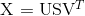1. 由于很多词没有出现，导致矩阵极其稀疏，因此需要对词频做额外处理来达到好的矩阵分解效果；

2. 矩阵非常大，维度太高(通常达到$10^6 \times 10^6$的数量级)；

3. 需要手动去掉停用词（如although, a,...），不然这些频繁出现的词也会影响矩阵分解的效果。

## 效果展示¶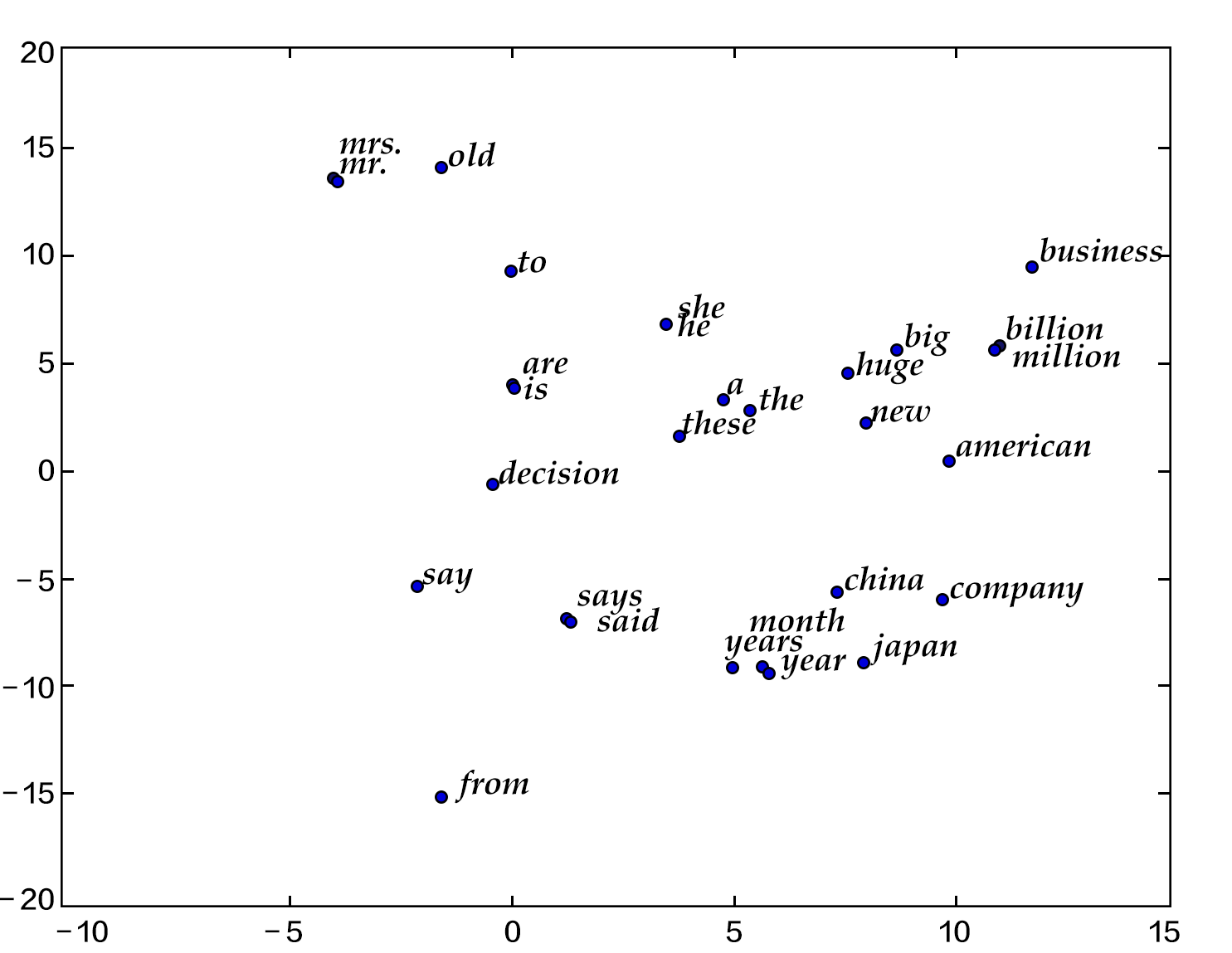please input two words: big huge
similarity: 0.899180685161

please input two words: from company
similarity: -0.0997506977351


## 模型概览¶

### 语言模型¶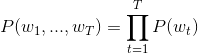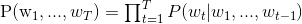### N-gram neural model¶

Yoshua Bengio等科学家就于2003年在著名论文 Neural Probabilistic Language Models  中介绍如何学习一个神经元网络表示的词向量模型。文中的神经概率语言模型（Neural Network Language Model，NNLM）通过一个线性映射和一个非线性隐层连接，同时学习了语言模型和词向量，即通过学习大量语料得到词语的向量表达，通过这些向量得到整个句子的概率。因所有的词语都用一个低维向量来表示，用这种方法学习语言模型可以克服维度灾难（curse of dimensionality）。注意：由于“神经概率语言模型”说法较为泛泛，我们在这里不用其NNLM的本名，考虑到其具体做法，本文中称该模型为N-gram neural model。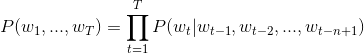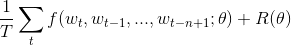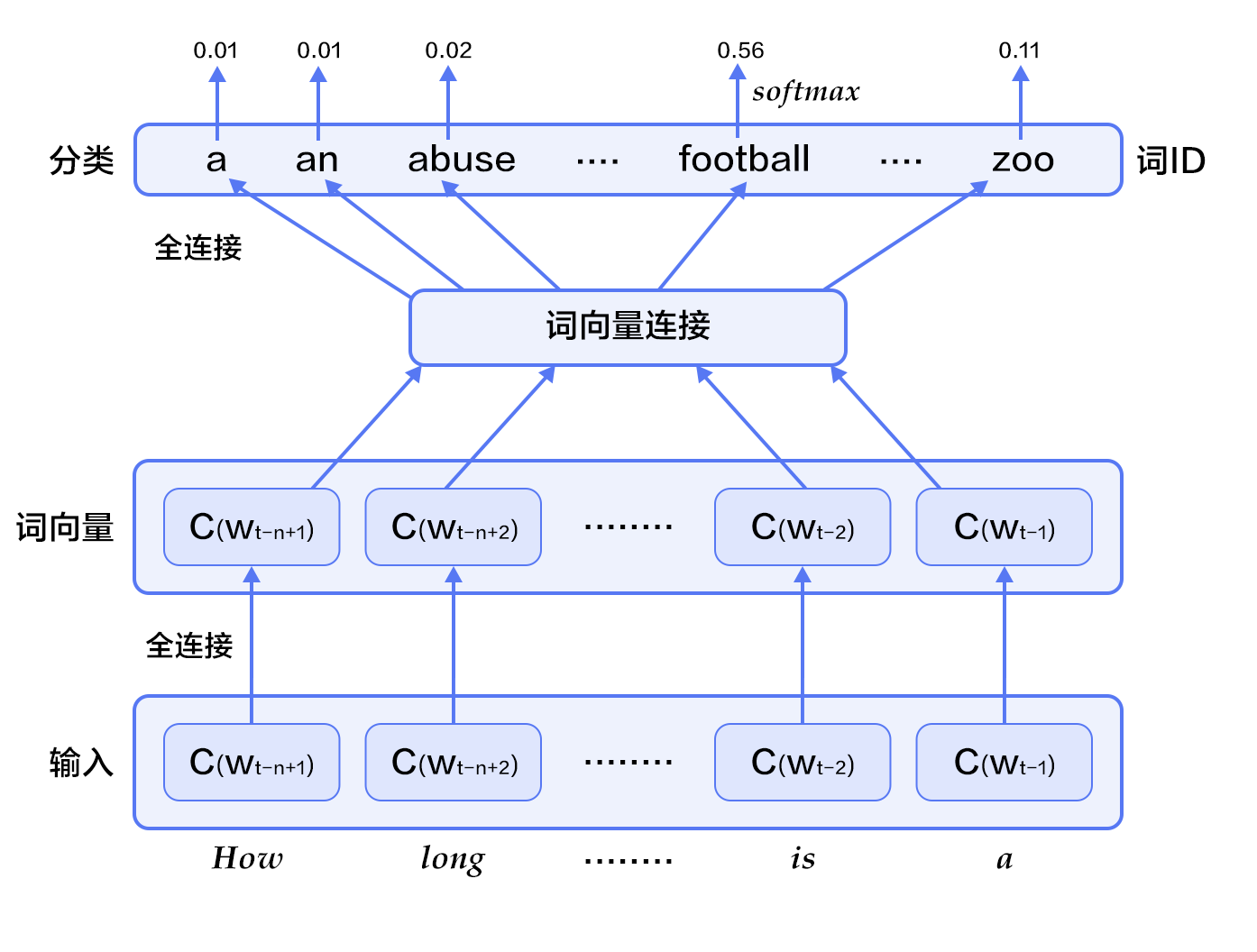• 对于每个样本，模型输入$w_{t-n+1},...w_{t-1}$, 输出句子第t个词在字典中|V|个词上的概率分布。

每个输入词$w_{t-n+1},...w_{t-1}$首先通过映射矩阵映射到词向量$C(w_{t-n+1}),...C(w_{t-1})$。

• 然后所有词语的词向量拼接成一个大向量，并经过一个非线性映射得到历史词语的隐层表示：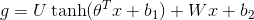其中，$x$为所有词语的词向量拼接成的大向量，表示文本历史特征；$\theta$、$U$、$b_1$、$b_2$和$W$分别为词向量层到隐层连接的参数。$g$表示未经归一化的所有输出单词概率，$g_i$表示未经归一化的字典中第$i$个单词的输出概率。

• 根据softmax的定义，通过归一化$g_i$, 生成目标词$w_t$的概率为：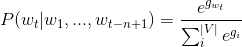• 整个网络的损失值(cost)为多类分类交叉熵，用公式表示为

<p align="center">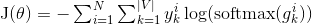### Continuous Bag-of-Words model(CBOW)¶

CBOW模型通过一个词的上下文（各N个词）预测当前词。当N=2时，模型如下图所示：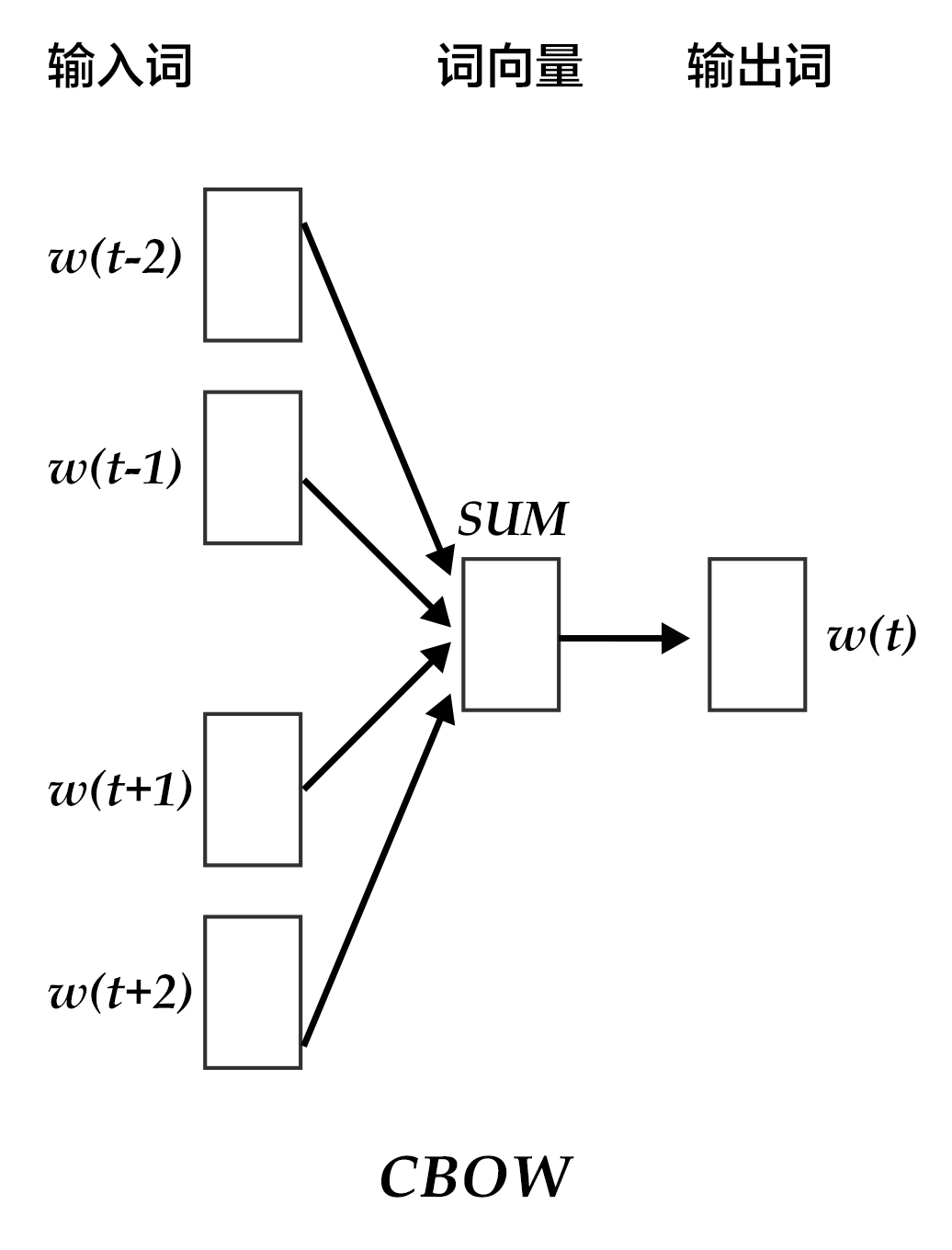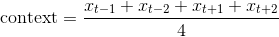### Skip-gram model¶

CBOW的好处是对上下文词语的分布在词向量上进行了平滑，去掉了噪声，因此在小数据集上很有效。而Skip-gram的方法中，用一个词预测其上下文，得到了当前词上下文的很多样本，因此可用于更大的数据集。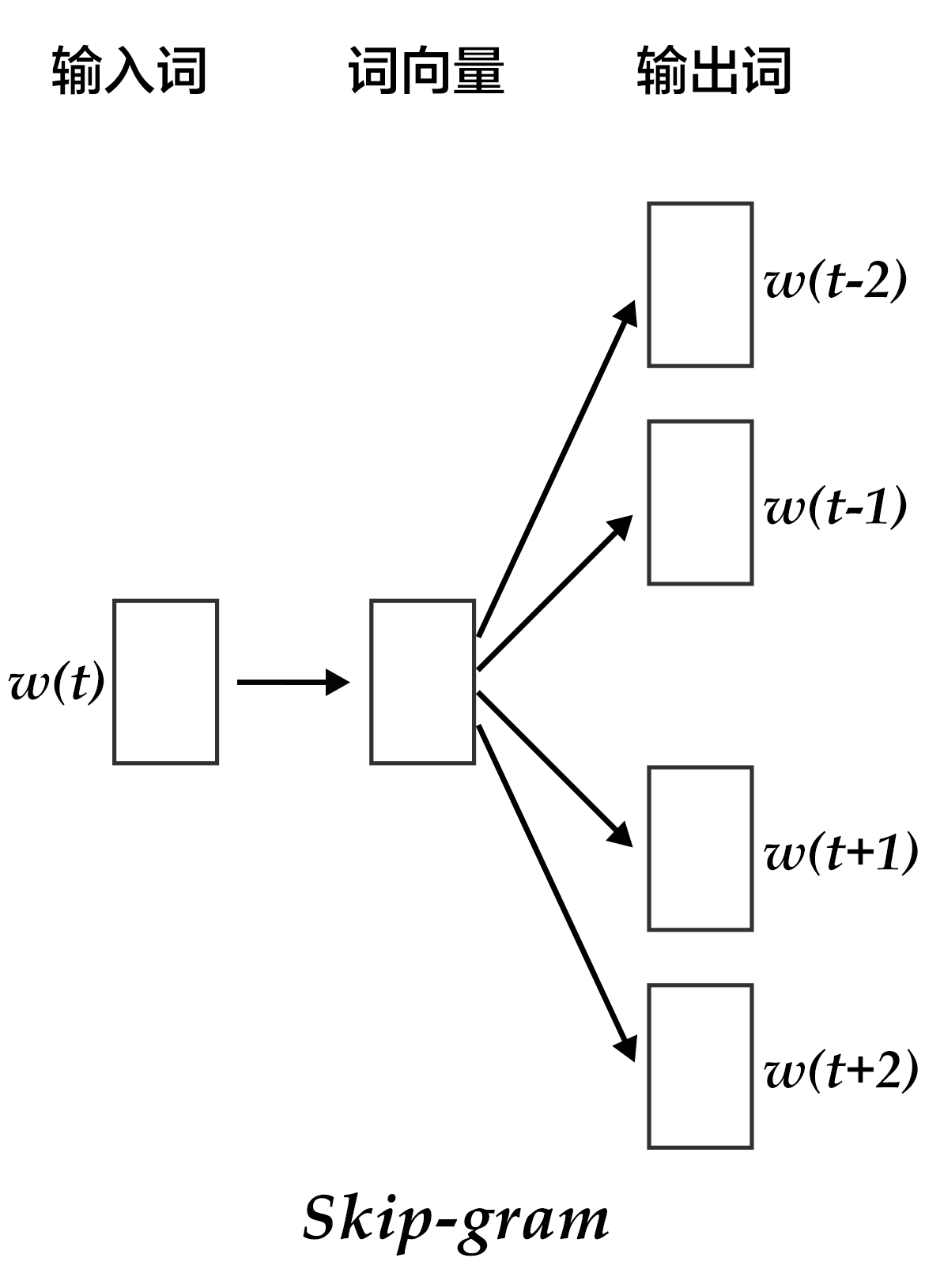## 数据准备¶

### 数据介绍¶

 训练数据 验证数据 测试数据 ptb.train.txt ptb.valid.txt ptb.test.txt 42068句 3370句 3761句

### 数据预处理¶

<s> I have a dream
I have a dream that
have a dream that one
a dream that one day
dream that one day <e>


## 编程实现¶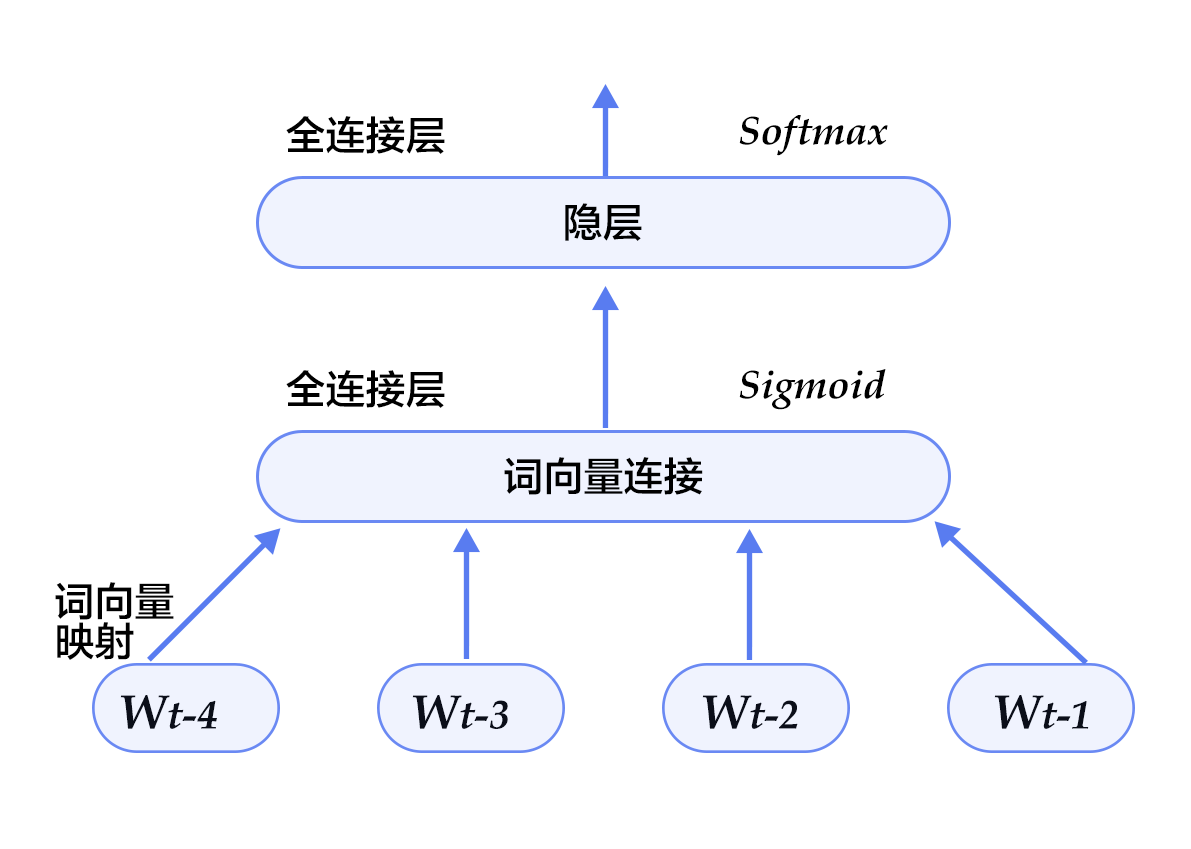from __future__ import print_function

import six
import numpy
import math


EMBED_SIZE = 32      # embedding维度
HIDDEN_SIZE = 256    # 隐层大小
N = 5                # ngram大小，这里固定取5
BATCH_SIZE = 100     # batch大小
PASS_NUM = 100       # 训练轮数

use_cuda = False  # 如果用GPU训练，则设置为True

dict_size = len(word_dict)


• 我们来定义我们的 N-gram 神经网络结构。这个结构在训练和预测中都会使用到。因为词向量比较稀疏，我们传入参数 is_sparse == True, 可以加速稀疏矩阵的更新。

def inference_program(words, is_sparse):

embed_first = fluid.embedding(
input=words,
size=[dict_size, EMBED_SIZE],
dtype='float32',
is_sparse=is_sparse,
param_attr='shared_w')
embed_second = fluid.embedding(
input=words,
size=[dict_size, EMBED_SIZE],
dtype='float32',
is_sparse=is_sparse,
param_attr='shared_w')
embed_third = fluid.embedding(
input=words,
size=[dict_size, EMBED_SIZE],
dtype='float32',
is_sparse=is_sparse,
param_attr='shared_w')
embed_fourth = fluid.embedding(
input=words,
size=[dict_size, EMBED_SIZE],
dtype='float32',
is_sparse=is_sparse,
param_attr='shared_w')

concat_embed = fluid.layers.concat(
input=[embed_first, embed_second, embed_third, embed_fourth], axis=1)
hidden1 = fluid.layers.fc(input=concat_embed,
size=HIDDEN_SIZE,
act='sigmoid')
predict_word = fluid.layers.fc(input=hidden1, size=dict_size, act='softmax')
return predict_word

• 基于以上的神经网络结构，我们可以如下定义我们的训练方法

def train_program(predict_word):
# 'next_word'的定义必须要在inference_program的声明之后，
# 否则train program输入数据的顺序就变成了[next_word, firstw, secondw,
# thirdw, fourthw], 这是不正确的.
next_word = fluid.data(name='nextw', shape=[None, 1], dtype='int64')
cost = fluid.layers.cross_entropy(input=predict_word, label=next_word)
avg_cost = fluid.layers.mean(cost)
return avg_cost

def optimizer_func():
learning_rate=3e-3,
regularization=fluid.regularizer.L2DecayRegularizer(8e-4))

• 现在我们可以开始训练啦。如今的版本较之以前就简单了许多。我们有现成的训练和测试集：paddle.dataset.imikolov.train()paddle.dataset.imikolov.test()。两者都会返回一个读取器。在PaddlePaddle中，读取器是一个Python的函数，每次调用，会读取下一条数据。它是一个Python的generator。

paddle.batch 会读入一个读取器，然后输出一个批次化了的读取器。我们还可以在训练过程中输出每个步骤，批次的训练情况。

def train(if_use_cuda, params_dirname, is_sparse=True):
place = fluid.CUDAPlace(0) if if_use_cuda else fluid.CPUPlace()

first_word = fluid.data(name='firstw', shape=[None, 1], dtype='int64')
second_word = fluid.data(name='secondw', shape=[None, 1], dtype='int64')
third_word = fluid.data(name='thirdw', shape=[None, 1], dtype='int64')
forth_word = fluid.data(name='fourthw', shape=[None, 1], dtype='int64')
next_word = fluid.data(name='nextw', shape=[None, 1], dtype='int64')

word_list = [first_word, second_word, third_word, forth_word, next_word]
feed_order = ['firstw', 'secondw', 'thirdw', 'fourthw', 'nextw']

main_program = fluid.default_main_program()
star_program = fluid.default_startup_program()

predict_word = inference_program(word_list, is_sparse)
avg_cost = train_program(predict_word)
test_program = main_program.clone(for_test=True)

optimizer = optimizer_func()
optimizer.minimize(avg_cost)

exe = fluid.Executor(place)

count = 0
feed_var_list = [
program.global_block().var(var_name) for var_name in feed_order
]
feeder_test = fluid.DataFeeder(feed_list=feed_var_list, place=place)
test_exe = fluid.Executor(place)
accumulated = len([avg_cost]) * 
avg_cost_np = test_exe.run(
program=program,
feed=feeder_test.feed(test_data),
fetch_list=[avg_cost])
accumulated = [
x + x for x in zip(accumulated, avg_cost_np)
]
count += 1
return [x / count for x in accumulated]

def train_loop():
step = 0
feed_var_list_loop = [
main_program.global_block().var(var_name) for var_name in feed_order
]
feeder = fluid.DataFeeder(feed_list=feed_var_list_loop, place=place)
exe.run(star_program)
for pass_id in range(PASS_NUM):
avg_cost_np = exe.run(
main_program, feed=feeder.feed(data), fetch_list=[avg_cost])

if step % 10 == 0:

print("Step %d: Average Cost %f" % (step, outs))

# 整个训练过程要花费几个小时，如果平均损失低于5.8，
# 我们就认为模型已经达到很好的效果可以停止训练了。
# 注意5.8是一个相对较高的值，为了获取更好的模型，可以将
# 这里的阈值设为3.5，但训练时间也会更长。
if outs < 5.8:
if params_dirname is not None:
fluid.io.save_inference_model(params_dirname, [
'firstw', 'secondw', 'thirdw', 'fourthw'
], [predict_word], exe)
return
step += 1
if math.isnan(float(avg_cost_np)):
sys.exit("got NaN loss, training failed.")

raise AssertionError("Cost is too large {0:2.2}".format(avg_cost_np))

train_loop()

• train_loop将会开始训练。期间打印训练过程的日志如下：

Step 0: Average Cost 7.337213
Step 10: Average Cost 6.136128
Step 20: Average Cost 5.766995
...


## 模型应用¶

### 预测下一个词¶

def infer(use_cuda, params_dirname=None):
place = fluid.CUDAPlace(0) if use_cuda else fluid.CPUPlace()

exe = fluid.Executor(place)

inference_scope = fluid.core.Scope()
with fluid.scope_guard(inference_scope):
# feed变量的名称feed_target_names和从scope中fetch的对象fetch_targets
[inferencer, feed_target_names,

# 设置输入，用四个LoDTensor来表示4个词语。这里每个词都是一个id，
# 用来查询embedding表获取对应的词向量，因此其形状大小是。
# recursive_sequence_lengths设置的是基于长度的LoD，因此都应该设为[]
# 注意recursive_sequence_lengths是列表的列表
data1 = numpy.asarray([], dtype=numpy.int64)  # 'among'
data2 = numpy.asarray([], dtype=numpy.int64)  # 'a'
data3 = numpy.asarray([], dtype=numpy.int64)  # 'group'
data4 = numpy.asarray([], dtype=numpy.int64)  # 'of'
lod = numpy.asarray([], dtype=numpy.int64)

first_word = fluid.create_lod_tensor(data1, lod, place)
second_word = fluid.create_lod_tensor(data2, lod, place)
third_word = fluid.create_lod_tensor(data3, lod, place)
fourth_word = fluid.create_lod_tensor(data4, lod, place)

assert feed_target_names == 'firstw'
assert feed_target_names == 'secondw'
assert feed_target_names == 'thirdw'
assert feed_target_names == 'fourthw'

# 构造feed词典 {feed_target_name: feed_target_data}
# 预测结果包含在results之中
results = exe.run(
inferencer,
feed={
feed_target_names: first_word,
feed_target_names: second_word,
feed_target_names: third_word,
feed_target_names: fourth_word
},
fetch_list=fetch_targets,
return_numpy=False)

print(numpy.array(results))
most_possible_word_index = numpy.argmax(results)
print(most_possible_word_index)
print([
key for key, value in six.iteritems(word_dict)
if value == most_possible_word_index
])


[[0.03768077 0.03463154 0.00018074 ... 0.00022283 0.00029888 0.02967956]]
0
the


def main(use_cuda, is_sparse):
if use_cuda and not fluid.core.is_compiled_with_cuda():
return

params_dirname = "word2vec.inference.model"

train(
if_use_cuda=use_cuda,
params_dirname=params_dirname,
is_sparse=is_sparse)

infer(use_cuda=use_cuda, params_dirname=params_dirname)

main(use_cuda=use_cuda, is_sparse=True)


## 参考文献¶

1. Bengio Y, Ducharme R, Vincent P, et al. A neural probabilistic language model[J]. journal of machine learning research, 2003, 3(Feb): 1137-1155.

2. Mikolov T, Kombrink S, Deoras A, et al. Rnnlm-recurrent neural network language modeling toolkit[C]//Proc. of the 2011 ASRU Workshop. 2011: 196-201.

3. Mikolov T, Chen K, Corrado G, et al. Efficient estimation of word representations in vector space[J]. arXiv preprint arXiv:1301.3781, 2013.

4. Maaten L, Hinton G. Visualizing data using t-SNE[J]. Journal of Machine Learning Research, 2008, 9(Nov): 2579-2605.

5. https://en.wikipedia.org/wiki/Singular_value_decomposition